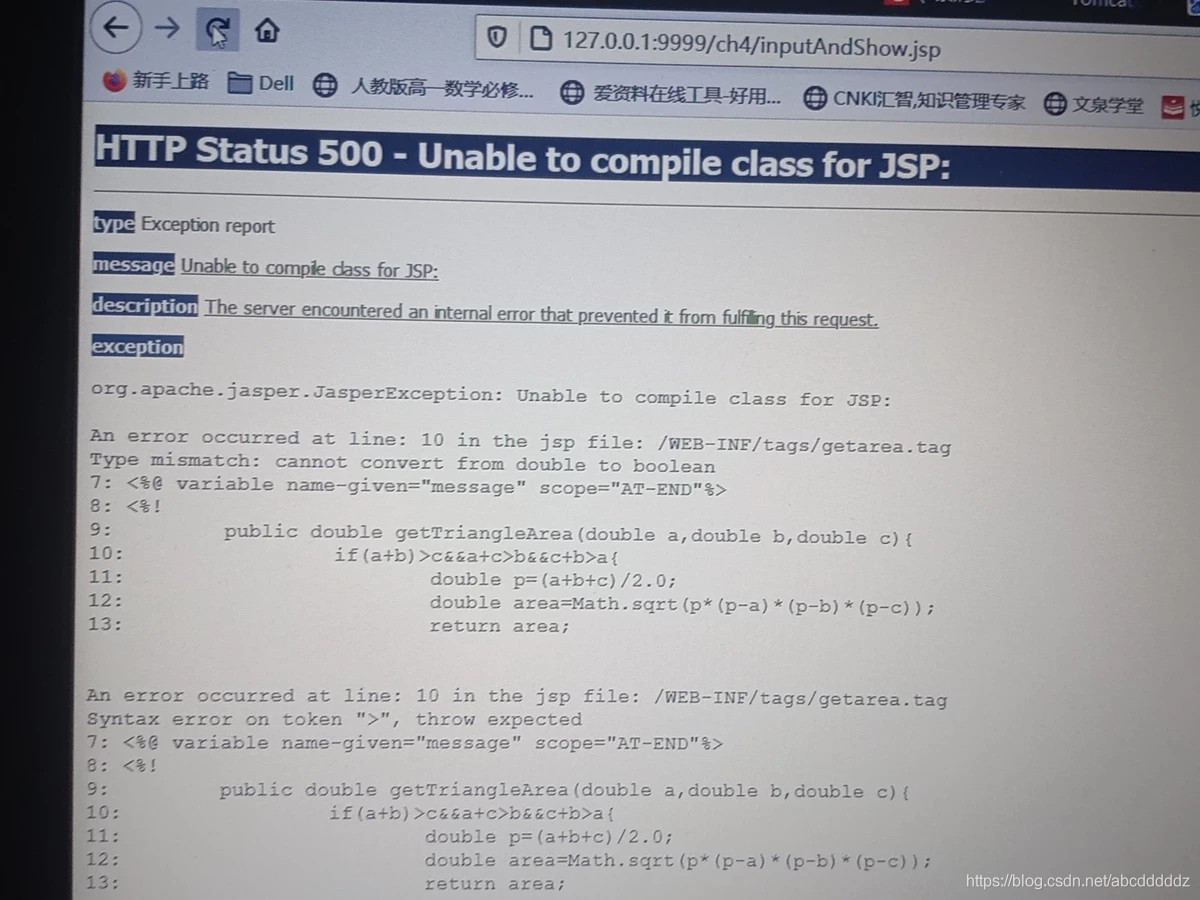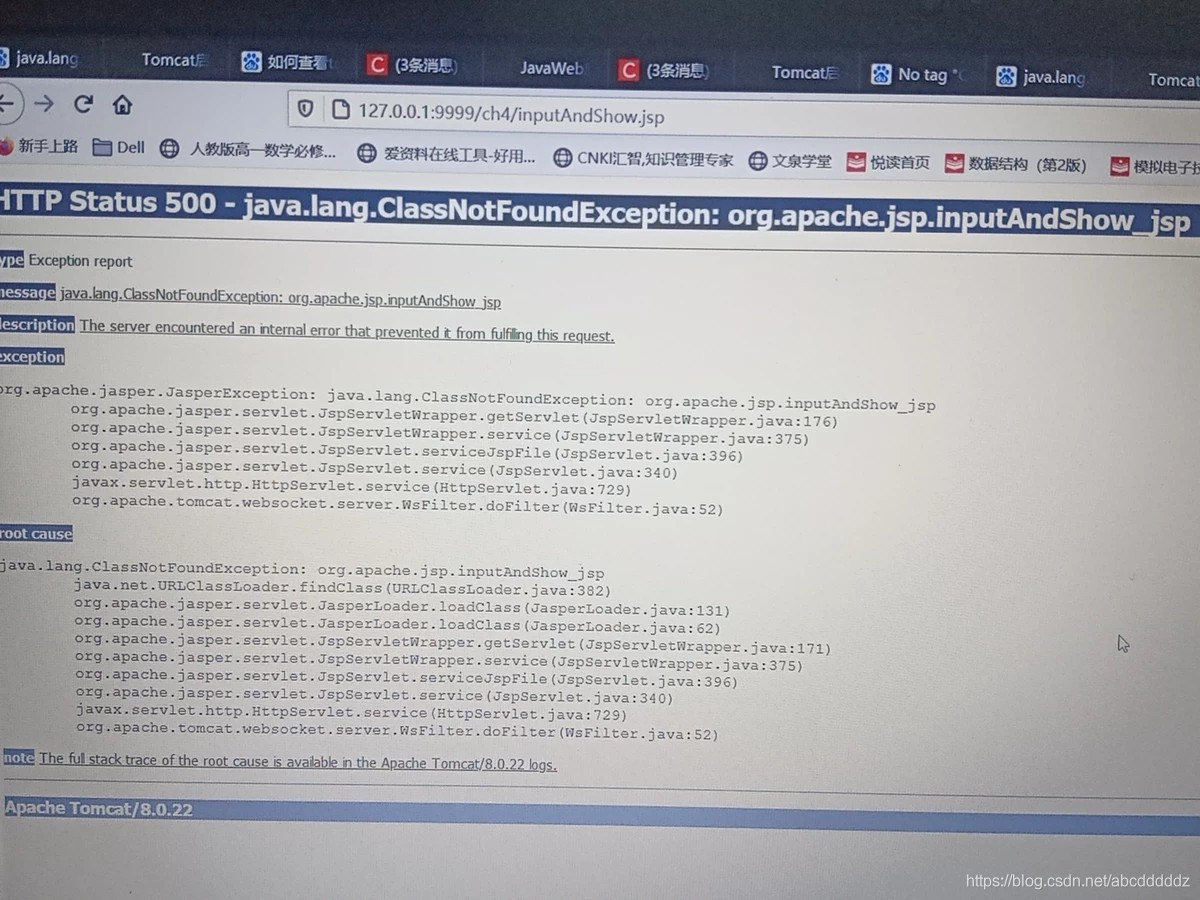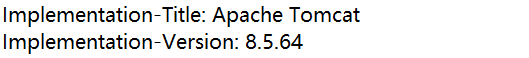# Tomcat error: 500 The server encountered an internal error that prevented it from fulfilling this request

Background:
I use JDK1.8 and tomcat8.0, but I encounter the following two problemsthen I made a new tomcat, and its version is as follows:and it succeeded…..

The reason: Tomcat version is higher than JDK version

In addition, I have problems with my code
here is the error code:

``````<%@ page contentType="text/html;charset=UTF-8" %>
<%@ taglib tagdir="/WEB-INF/tags" prefix="computer"%>
<html>
The three values entered a,b,c (representing the three sides of a triangle or the upper base, lower base and height of a trapezoid);
<body color=cyan>
<form action="" method=get name=form>
<%--Get parameters to submit to yourself--%>
<table>
<tr><td>Enter value a: </td>
<td><input type="text" name="a"></td>
</tr>
<tr><td>input value b: </td>
<td><input type="text" name="b"></td>
</tr>
<tr><td>input value c: </td>
<td><input type="text" name="c"></td>
</tr>
</table>
<input type="radio" name="r" value="triangle">stands for triangle
<br>
<input type="submit" name="submit" value="submit" name=submit>stands for submit button
</form>
<% String a=request.getParameter("a");
String b=request.getParameter("b");
String c=request.getParameter("c");
String cd=request.getParameter("r");
if(a==null||b==null||c==null){
a="0";
b="0";
c="0";
cd="0";

}
if(a.length()>0&&b.length()>0&&c.length()>0){
%>
<computer:Getarea numberA="<%=a%>" numberB="<%=b%>"
numberC="<%=c%>" condition="<%=cd%>"/>

<br><%= message%>
<br><%= area %>

<% }
%>
</body>
</html>

``````

The reason for the error is that there are two more spaces. The error report is the same
here is the correct code:

``````<%@ page contentType="text/html;charset=UTF-8" %>
<%@ taglib tagdir="/WEB-INF/tags" prefix="computer"%>
<html>
The three values entered a,b,c (representing the three sides of a triangle or the upper base, lower base and height of a trapezoid);
<body color=cyan>
<form action="" method=get name=form>
<%--Get parameters to submit to yourself--%>
<table>
<tr><td>Enter value a: </td>
<td><input type="text" name="a"></td>
</tr>
<tr><td>input value b: </td>
<td><input type="text" name="b"></td>
</tr>
<tr><td>input value c: </td>
<td><input type="text" name="c"></td>
</tr>
</table>
<input type="radio" name="r" value="triangle">stands for triangle
<br>
<input type="submit" name="submit" value="submit" name=submit>stands for submit button
</form>
<% String a=request.getParameter("a");
String b=request.getParameter("b");
String c=request.getParameter("c");
String cd=request.getParameter("r");
if(a==null||b==null||c==null){
a="0";
b="0";
c="0";
cd="0";

}
if(a.length()>0&&b.length()>0&&c.length()>0){
%>
<computer:Getarea numberA="<%=a%>" numberB="<%=b%>"
numberC="<%=c%>" condition="<%=cd%>"/>

<br><%=message%>
<br><%=area %>

<% }
%>
</body>
</html>
``````